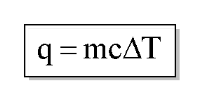# Problem: A 70.0 g piece of metal at 80.0°C is placed in 100 g of water at 22.0°C contained in a calorimeter like that shown in Figure 5.12. The metal and water come to the same temperature at 24.6°C. How much heat did the metal give up to the water? What is the specific heat of the metal?

###### FREE Expert Solution
• Amount of heat can be calculated using the equation:Where q is the amount of heat in J; c as specific heat in J/goC; m is mass in grams; and ΔT as change in temperature in oC

80% (394 ratings)###### Problem Details

A 70.0 g piece of metal at 80.0°C is placed in 100 g of water at 22.0°C contained in a calorimeter like that shown in Figure 5.12. The metal and water come to the same temperature at 24.6°C. How much heat did the metal give up to the water? What is the specific heat of the metal?